• +91 9971497814
• info@interviewmaterial.com

# RD Chapter 15- Areas Related to Circles Ex-15.1 Interview Questions Answers

### Related Subjects

Question 1 : Find the circumference and area of a circle of radius 4.2 cm.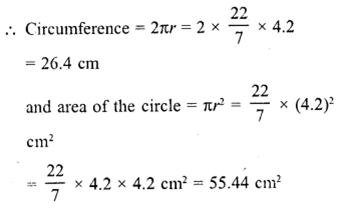Question 2 : Find the circumference of a circle whose area is 301.84 cm2.

Area of a circle = 301.84 cm2
Let r be the radius, then πr2 = 301.84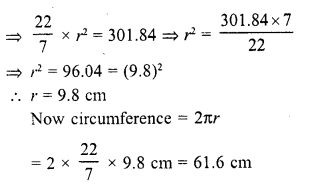Question 3 : Find the area of a circle whose circum¬ference is 44 cm.

Circumference of a circle = 44 cm
then 2πr = circumference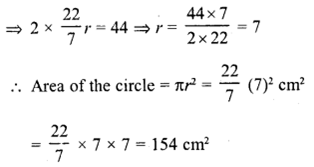Question 4 : The circumference of a circle exceeds the diameter by 16.8 cm. Find the circum¬ference of the circle. (C.B.S.E. 1996)

Let r be the radius of the circle
∴  Circumference = 2r + 16.8 cm
⇒  2πr = 2r + 16.8
⇒  2πr – 2r = 16.8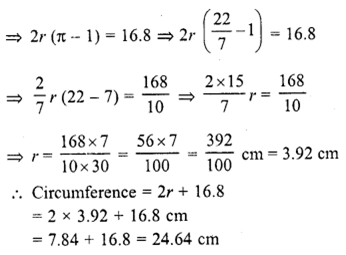Question 5 : A horse is tied to a pole with 28 m long string. Find the area where the horse can graze. (Take π = 22/7)

Radius of the circle (r) = Length of the rope = 28 m .
Area of the place where the horse can graze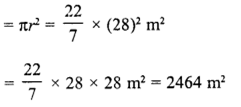Question 6 : A steel wire when bent in the form of a square encloses an area of 121 cm2. If the same wire is bent in the form of a circle, find the area of the circle.  (C.B.S.E. 1997)

Area of square formed by a wire =121 cm2
∴ Side of square (a) =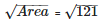= 11 cm Perimeter of the square = 4 x side = 4 x 11 = 44 cm
∴Circumference of the circle formed by the wire = 44cm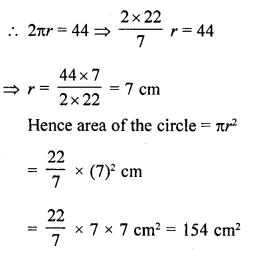Question 7 : The circumference of two circles are in the ratio 2 : 3. Find the ratio of their areas.

Answer 7 : Let R and r be the radii of two circles and their ratio between them circumference = 2 : 3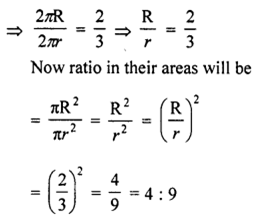Question 8 : The sum of radii of two circles is 140 cm and the difference of their circum¬ferences is 88 cm. Find the diameters of the circles.

Let R and r be the radii of two circles Then R + r = 140 cm  …….(i)
and difference of their circumferences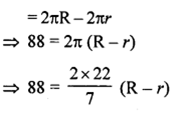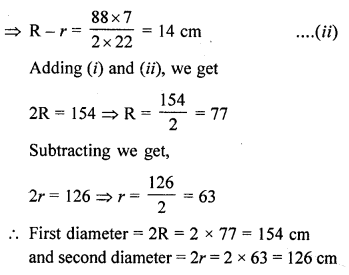Question 9 : Find the radius of a circle whose circumference is equal to the sum of the circumferences of two circles of radii 15 cm and 18 cm. [NCERT Exemplar]

Let the radius of a circle be r.
Circumference of a circle = 2πr
Let the radii of two circles are r1 andr2 whose
values are 15 cm and 18 cm respectively.
i.e., r1 = 15 cmand r2 = 18 cm
Now, by given condition,
Circumference of circle = Circumference of first circle + Circumference of secondcircle
2πr = 2π r1 + 2πr=
r = r1 + r2
r = 15 + 18
r = 33cm
Hence, required radius of a circle is 33 cm.

Question 10 : The radii of two circles are 8 cm and 6 cm respectively. Find the radius of the circle having its area equal to the sum of the areas of the two circles.

Radius of first circle (r1) = 8 cm
and radius of second circle (r2) = 6 cm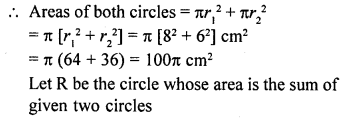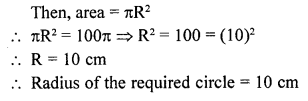Todays Deals### RD Chapter 15- Areas Related to Circles Ex-15.1 Contributorskrishan

Name:
Email:

# Latest News# 9000 interview questions in different categories